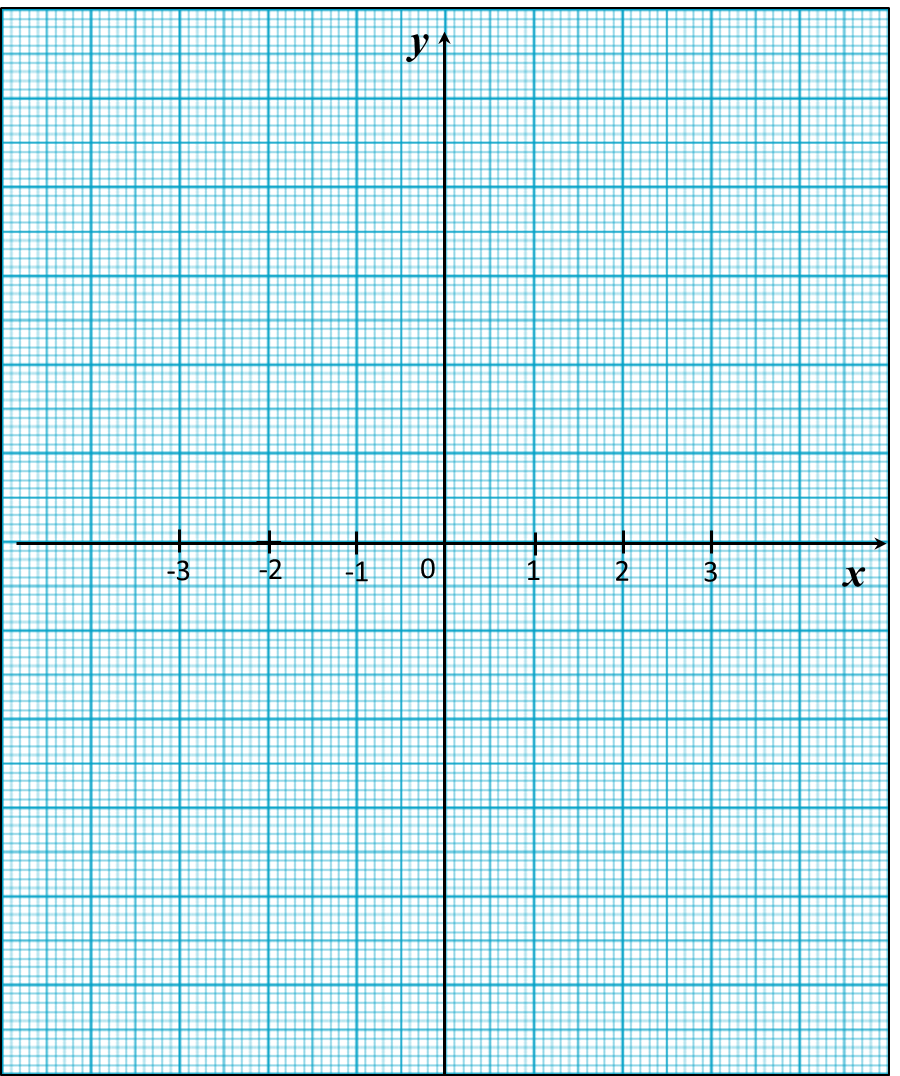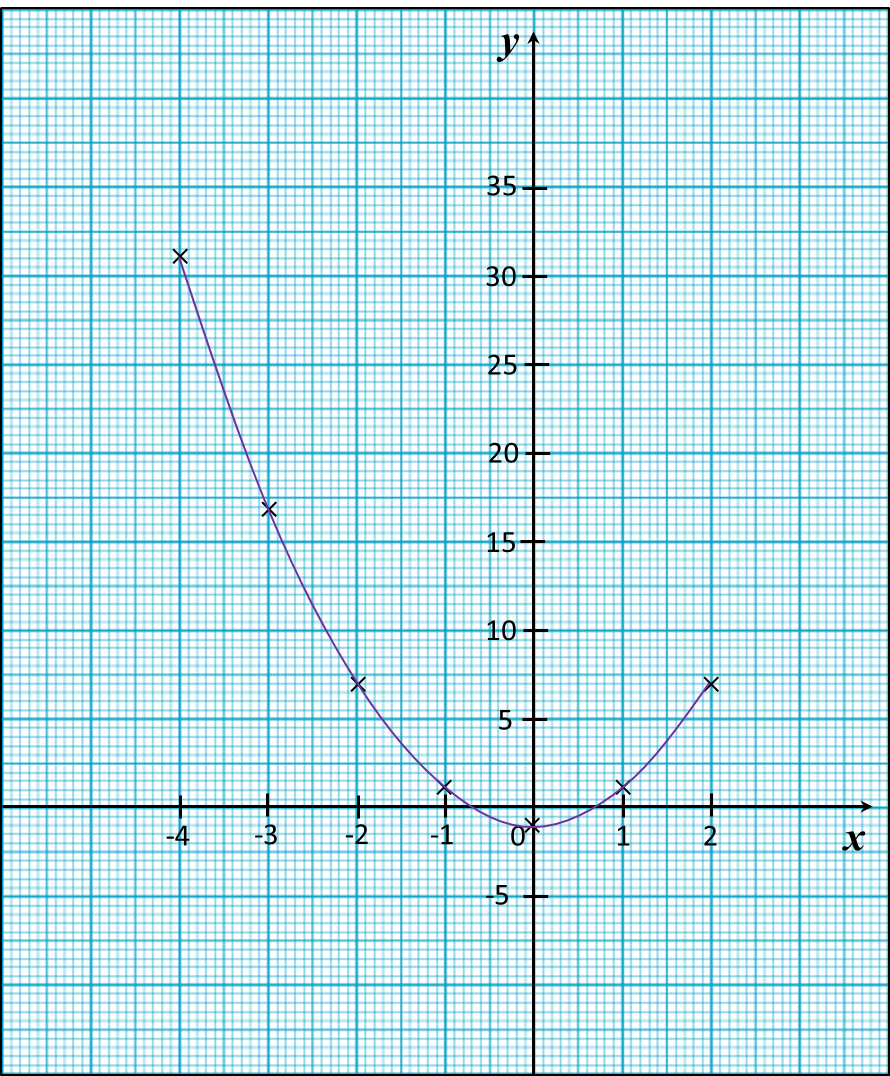# 13.2.2 Graphs of Functions, PT3 Focus Practice

 
 
13.2.2 Graphs of Functions, PT3 Focus Practice
 
 
Question 4:
 
Use graph paper to answer this question.
 
Table below shows the values of two variables, x and y, of a function.
 

 
 
   x     –3     –2     –1     0     1     2     3     y     –19     –3     1     –1     –3     1     17  
 
 
The x-axis and the y-axis are provided on the graph paper on the answer space.
 
(a)  By using a scale of 2 cm to 5 units, complete and label the y-axis.
 
(b) Based on the table above, plot the points on the graph paper.
 
(c)  Hence, draw the graph of the function.
 
 

 Answer:Solution:Question 5:
 
Use graph paper to answer this question.
 
Table below shows the values of two variables, x and y, of a function.
 

 
 
   x     –4     –3     –2     –1     0     1     2     y     31     17     7     1     –1     1     7  
 
 
The x-axis and the y-axis are provided on the graph paper on the answer space.
 
(a)  By using a scale of 2 cm to 5 units, complete and label the y-axis.
 
(b)   Based on the table above, plot the points on the graph paper.
 
(c)  Hence, draw the graph of the function.Solution:Question 6:
(a) Complete table below in the answer space for the equation L = x2 + 5x by writing the value of L when x = 2.
(b) Use graph paper to answer this part of the question. You may use a flexible curve rule.
By using a scale of 2 cm to 1 unit on the x-axis and 2 cm to 5 units on the y-axis, draw the graph of L = x2 + 5x for 0 ≤ x ≤ 4.